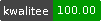++ed by:
1 non-PAUSE user
and 1 contributors

# NAME

LCS - Longest Common Subsequence# SYNOPSIS

``````  use LCS;
my \$lcs = LCS->LCS( [qw(a b)], [qw(a b b)] );

# \$lcs now contains an arrayref of matching positions
# same as
\$lcs = [
[ 0, 0 ],
[ 1, 2 ]
];

my \$all_lcs = LCS->allLCS( [qw(a b)], [qw(a b b)] );

# same as
\$all_lcs = [
[
[ 0, 0 ],
[ 1, 1 ]
],
[
[ 0, 0 ],
[ 1, 2 ]
]
];``````

# DESCRIPTION

LCS is an implementation based on the traditional LCS algorithm.

It contains reference implementations working slow but correct.

Also some utility methods are added to reformat the result.

## CONSTRUCTOR

new()

Creates a new object which maintains internal storage areas for the LCS computation. Use one of these per concurrent LCS() call.

## METHODS

LCS(\@a,\@b)

Finds a Longest Common Subsequence, taking two arrayrefs as method arguments. It returns an array reference of corresponding indices, which are represented by 2-element array refs.

``````  # position  0 1 2
my \$a = [qw(a b  )];
my \$b = [qw(a b b)];

my \$lcs = LCS->LCS(\$a,\$b);

# same like
\$lcs = [
[ 0, 0 ],
[ 1, 1 ]
];``````
LLCS(\@a,\@b)

Calculates the length of the Longest Common Subsequence.

``````  my \$llcs = LCS->LLCS( [qw(a b)], [qw(a b b)] );
print \$llcs,"\n";   # prints 2

# is the same as
\$llcs = scalar @{LCS->LCS( [qw(a b)], [qw(a b b)] )};``````
allLCS(\@a,\@b)

Finds all Longest Common Subsequences. It returns an array reference of all LCS.

``````  my \$all_lcs = LCS->allLCS( [qw(a b)], [qw(a b b)] );

# same as
\$all_lcs = [
[
[ 0, 0 ],
[ 1, 1 ]
],
[
[ 0, 0 ],
[ 1, 2 ]
]
];``````

The purpose is mainly for testing LCS algorithms, as they only return one of the optimal solutions. If we want to know, that the result is one of the optimal solutions, we need to test, if the solution is part of all optimal LCS:

``````  use Test::More;
use Test::Deep;
use LCS;
use LCS::Tiny;

cmp_deeply(
LCS::Tiny->LCS(\@a,\@b),
any(@{LCS->allLCS(\@a,\@b)} ),
"Tiny::LCS \$a, \$b"
);``````
lcs2align(\@a,\@b,\$LCS)

Returns the two sequences aligned, missing positions are represented as empty strings.

``````  use Data::Dumper;
use LCS;
print Dumper(
LCS->lcs2align(
[qw(a   b)],
[qw(a b b)],
LCS->LCS([qw(a b)],[qw(a b b)])
)
);
# prints

\$VAR1 = [
[
'a',
'a'
],
[
'',
'b'
],
[
'b',
'b'
]
];``````
align(\@a,\@b)

Returns the same as lcs2align() via calling LCS() itself.

sequences2hunks(\$a, \$b)

Transforms two array references of scalars to an array of hunks (two element arrays).

hunks2sequences(\$hunks)

Transforms an array of hunks to two arrays of scalars.

``````  use Data::Dumper;
use LCS;
print Dumper(
LCS->hunks2sequences(
LCS->LCS([qw(a b)],[qw(a b b)])
)
);
# prints (reformatted)
\$VAR1 = [ 0, 1 ];
\$VAR2 = [ 0, 2 ];``````
align2strings(\$hunks, \$gap_character)

Returns two strings aligned with gap characters. The default gap character is '_'.

``````  use Data::Dumper;
use LCS;
print Dumper(
LCS->align2strings(
LCS->lcs2align([qw(a b)],[qw(a b b)],LCS->LCS([qw(a b)],[qw(a b b)]))
)
);
\$VAR1 = 'a_b';
\$VAR2 = 'abb';``````
fill_strings(\$string1, \$string2, \$fill_character)

Returns both strings filling up the shorter with \$fill_character to the same length.

The default \$fill_character is '_'.

clcs2lcs(\$compact_lcs)

Convert compact LCS to LCS.

lcs2clcs(\$compact_lcs)

Convert LCS to compact LCS.

max(\$i, \$j)

Returns the maximum of two numbers.

None by design.

# STABILITY

Until release of version 1.00 the included methods, names of methods and their interfaces are subject to change.

Beginning with version 1.00 the specification will be stable, i.e. not changed between major versions.

Ronald I. Greenberg. Fast and Simple Computation of All Longest Common Subsequences, http://arxiv.org/pdf/cs/0211001.pdf

Robert A. Wagner and Michael J. Fischer. The string-to-string correction problem. Journal of the ACM, 21(1):168-173, 1974.

# SOURCE REPOSITORY

http://github.com/wollmers/LCS

# AUTHOR

Helmut Wollmersdorfer <helmut.wollmersdorfer@gmail.com>Copyright 2014- Helmut Wollmersdorfer

# LICENSE

This library is free software; you can redistribute it and/or modify it under the same terms as Perl itself.